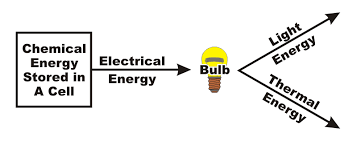# Energy Transfers And Transformations Mrc

Approved & Edited by ProProfs Editorial Team
At ProProfs Quizzes, our dedicated in-house team of experts takes pride in their work. With a sharp eye for detail, they meticulously review each quiz. This ensures that every quiz, taken by over 100 million users, meets our standards of accuracy, clarity, and engagement.
| Written by CB
C
CB
Community Contributor
Quizzes Created: 6 | Total Attempts: 6,018
Questions: 5 | Attempts: 892Settings.

• 1.

### Is the following statement TRUE or FALSE? Energy is never lost. It is only transferred or transformed.

• A.

True

• B.

False

A. True
Explanation
Energy is never lost because of the principle of conservation of energy, which states that energy cannot be created or destroyed, only transferred or transformed from one form to another. This means that even though energy may change from one form to another, the total amount of energy in a closed system remains constant. Therefore, the statement is true.

Rate this question:

• 2.

### What is the MAIN form of Energy the skater has at POINT A in the Diagram?

• A.

Gravitational Potential Energy

• B.

Elastic Potential Energy

• C.

Thermal Energy

• D.

Sound Energy

A. Gravitational Potential Energy
Explanation
At Point A in the diagram, the skater is at a height above the ground. Gravitational potential energy is the energy possessed by an object due to its position in a gravitational field. Since the skater is at a height, they have gravitational potential energy, which is the main form of energy at Point A. Elastic potential energy is associated with the stretching or compressing of an object, thermal energy is related to the heat of an object, and sound energy is produced by vibrations. None of these forms of energy are relevant to the skater at Point A.

Rate this question:

• 3.

### What is the Energy transformation that occurs as the skater moves from POINT A to POINT B on the diagram"

• A.

GPE to Kinetic Energy

• B.

Kinetic Energy to GPE

• C.

Elastic Potential Energy to Kinetic Energy

• D.

GPE to Thermal Energy

A. GPE to Kinetic Energy
Explanation
As the skater moves from POINT A to POINT B on the diagram, the energy transformation that occurs is from gravitational potential energy (GPE) to kinetic energy. Initially, at POINT A, the skater has a certain height and therefore possesses GPE. As the skater moves downwards towards POINT B, the GPE is converted into kinetic energy, which is the energy of motion. This transformation occurs due to the force of gravity acting on the skater, causing them to accelerate and gain speed as they descend.

Rate this question:

• 4.

### Which is the correct statement below?

• A.

At POINT C the skater has LESS Kinetic energy but MORE GPE than at POINT B

• B.

At POINT C the skater has MORE Kinetic energy but LESS GPE than at POINT B

A. At POINT C the skater has LESS Kinetic energy but MORE GPE than at POINT B
Explanation
At Point C, the skater has less kinetic energy because kinetic energy is dependent on the speed of the skater, and at Point C the skater is moving slower compared to Point B. On the other hand, the skater has more gravitational potential energy (GPE) at Point C because GPE is dependent on the height of the skater, and at Point C the skater is at a higher elevation compared to Point B.

Rate this question:

• 5.

### What is the energy transformation that occurs in the Toaster Diagram shown?

• A.

Electrical Energy to Heat, kinetic and sound energy

• B.

Electrical Energy to kinetic and sound energy

• C.

Kinetic energy, heat and sound energy to Electrical EnergyBack to top